# Sort a Linked List of 0s,1s, and 2s

## Introduction

In this blog, we will discuss a problem that has been frequently asked in interviews.

The problem is to sort a linked list of 0s,1s, and 2s.

To understand the solution to the problem, we should first understand the concept of a Linked list.

linked list is a linear data structure consisting of nodes where each node points to the next node using a pointer. Each node is composed of data and a pointer to the next node.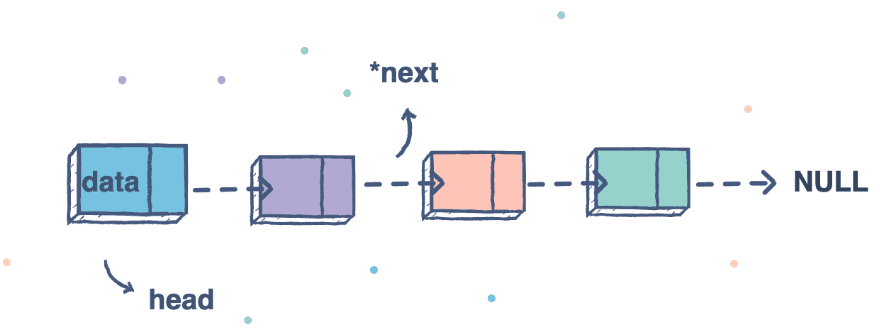Structure of a Node in a linked list:

``````struct Node
{
int key;
Node(int x){
key=x;
next=NULL;
}
struct Node *next;
};``````

To sort a linked list of 0s,1s, and 2s, we need to return the head of the linked list.

Some Examples

Sample Input 1: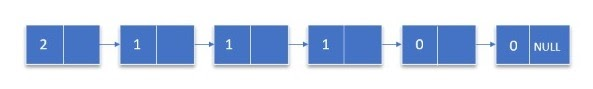Sample Output 1: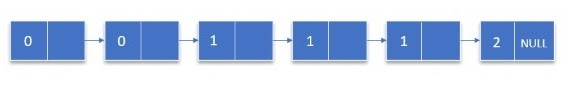Sample Input 2: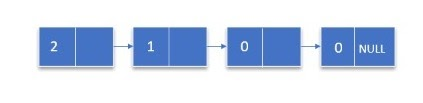Sample Output 2: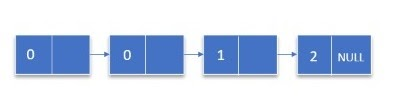## Approach

1. Create a function sort() that takes the linked list's head as a parameter and then traverses the linked list to count the 0s,1s, and 2s and stores these values in variables.
2. Let n1 contain the number of ones, n2 have the number of two's, and n3 includes the number of three's. We will fill the list with 0s,1s, and 2s till n1>0, n2>0, n3>0, respectively.
3. Since the linked list is now sorted, we will return the head of the linked list.

### Code In C++

``````// Sort a Linked List of 0s,1s, and 2s
#include <bits/stdc++.h>
using namespace std;

struct Node
{
int val;
Node(int x)
{
val = x; // variable to store value of node
next = NULL; // pointer to store address of next node
}
struct Node *next;
};

// Function to sort a linked list of 0s, 1s and 2s
{
int n0 = 0, n1 = 0, n2 = 0; // The variables to store the frequency of 0s, 1s and 2s

//Storing the count of 0,1 and 2 in the respective variables
while (curr != NULL)
{
if (curr->val == 0)
{
n0++; // Increasing frequency of 0s
}
else if (curr->val == 1)
{
n1++; // Increasing frequency of 1s
}
else if (curr->val == 2)
{
n2++; // Increasing frequency of 2s
}
curr = curr->next; // Traversing the linked list till end
}

//Since we have to traverse from the head, we are reinitializing the value of curr with head

while (curr != NULL)
{
if (n0 > 0) // filling the list with 0, till n0 > 0
{
curr->val = 0; // changing value of node to 0
curr = curr->next;
n0--;
}
else if (n1 > 0) // filling the list with 1, till n2 > 0
{
curr->val = 1; // changing value of node to 1
curr = curr->next;
n1--;
}
else if (n2 > 0) // filling the list with 2, till n2 > 0
{
curr->val = 2; // changing value of node to 2
curr = curr->next;
n2--;
}
}
}

// Function to insert a node
Node *insert(Node *x, int y)
{
Node *temp = new Node(y);
temp->next = x;
return temp;
}

// Function to print linked list
void printList(Node *node)
{
while (node != NULL)
{
cout << node->val << " ";
node = node->next;
}
cout << endl;
}

// Driver code
int main()
{
cout << "Input: ";

cout << "Output: ";
return 0;
}``````

Sample Input and Output:

``````Input:  0 2 2 1 1 1 0 2 0 1 0

Output: 0 0 0 0 1 1 1 1 2 2 2``````

### Complexity Analysis

Time Complexity: O(n) (Worst Case)

Since there are n nodes in the linked list and we are doing traversals of the whole linked list, therefore, the time complexity is O(n)

Space Complexity: O(1) (Worst case)

Since we are not using any data structures other than the linked list given to us, therefore, the space complexity is O(1)

### What is sorting?

Sorting is a technique that arranges a collection of data in a particular order; it can be ascending or descending.

### What are different types of linked lists?

There are three types of linked lists, namely:

### What are the applications of linked lists?

1. They are used in the Implementation of stacks and queues.
2. Linked lists are also used to implement graphs, i.e., Adjacency list representation of the graph.
3. Arithmetic operations on large integers are done using a linked list.

## Conclusion

In this article, we discussed how we could Sort a linked list of 0s,1s, and 2s. We discussed the optimized approach of sorting a linked list of 0s,1s, and 2s and its space and time complexity.

We hope you gained some insight into this problem, and now it is your turn to practice this problem and code it out in your way.

Suggested problems based on LinkedList are Count pairs from two linked lists whose sum is equal to a given value, Remove Duplicates from Sorted List, Add One to LinkedList, Cycle Detection in Singly LinkedList, and many more.

I hope that this blog has helped you enhance your knowledge regarding the above Data Structures and Algorithms problem and if you would like to learn more, check out our articles on the CodeStudio. Enroll in our courses, refer to the test series and problems available and look at the interview bundle for placement preparations.

Nevertheless, you may consider our paid courses to give your career an edge over others!

Do upvote our blogs if you find them helpful and engaging!

Until then, Keep Learning and Keep Coding.,